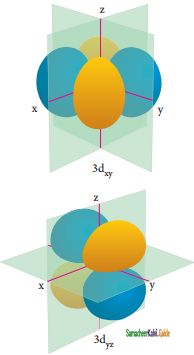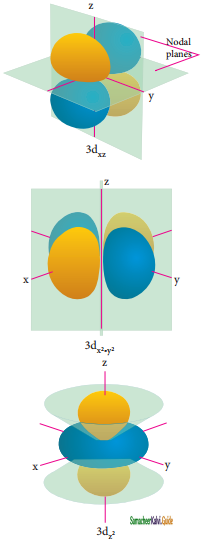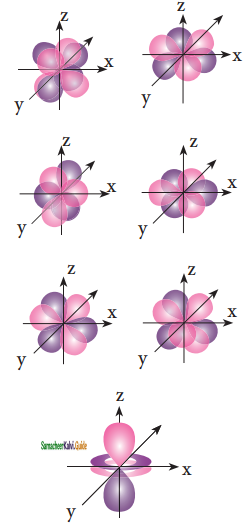Tamilnadu State Board New Syllabus Samacheer Kalvi 11th Chemistry Guide Pdf Chapter 2 Quantum Mechanical Model of Atom Text Book Back Questions and Answers, Notes.

## Tamilnadu Samacheer Kalvi 11th Chemistry Solutions Chapter 2 Quantum Mechanical Model of Atom

### 11th Chemistry Guide Quantum Mechanical Model of Atom Text Book Back Questions and Answers

Textual Questions:

I. Choose the best Answer:

Question 1.
Electronic configuration of species M2+ is 1s2 2s2 2p6 3s2 3p6 3d6 and its atomic weight is 56. The number of neutrons in the nucleus of species M is
(a) 26
(b) 22
(c) 30
(d) 24
(c) 30

Question 2.
The energy of light of wavelength 45nm is
(a) 6.65 × 1015 J
(b) 6.67 × 1011 J
(c) 4.42 × 10-18 J
(d) 4.42 × 10-5 V
(c) 4.42 × 10-18 J

Question 3.
The energies E1 and E2 of two radiation are 25 eV and 50 eV respectively. The radiation between their wavelengths ie., λ1 and λ2 will be
(a) $$\frac{\lambda_{1}}{\lambda_{2}}$$ = 1
(b) λ1 = 2 λ2
(c) λ1 = $$\sqrt{225 \times 50}$$ λ2
(d) 2λ1 = λ2
(b) λ1 = 2 λ2

Question 4.
Splitting of spectral lines in an electric field is called
(a) Zeeman effect
(b) shielding effect
(c) Compton effect
(d) stark effect
(d) stark effect

Question 5.
Based on equation E = -2.178 × 10-18 J(z2/n2), certain conclusions are written. Which of them is not correct?
(a) Equation can be used to calculate the change in energy when the electron changes orbit
(b) For n – 1 , the electron has a more negative energy then it does for n = 6 which means that the eiectron is more loosely bound in the smallest allowed orbit
(c) The negative sign in equation simply means that the energy of electron bound to the nucleus is lower than it would be if the electrons were at the infinite distance ffome nucleus.
(d) Larger the value of n, the larger is the orbit radius.
(b) For n – 1 , the electron has a more negative energy then it does for n = 6 which means that the eiectron is more loosely bound in the smallest allowed orbit.Question 6.
According to the Bohr Theory , which of the following transitions in the hydrogen atom will give rise to least energetic photon?
(a) n = 6 to n = 1
(b) n = 5 to n = 4
(c) n = 5 to n = 3
(d) n = 6 to n = 5
(d) n = 6 to n = 5

Question 7.
Assertion:
The spectrum of He+ is expected to be similar to that of hydrogen
Reason: He+ is also one electron system.
(a) If both assertion and reason are true and reason is the correct explanation of assertion.
(b) If both assertion and reason are true but reasons is not the correct explanation of assertion.
(c) If assertion is true but reason is false
(d) If both assertion and reason are false
(a) If both assertion and reason are true and reason is the correct explanation of assertion.

Question 8.
Which of the following pairs of d-orbitals will have electron density along the axes?
(a) dz2, dxz
(b) dxz, dyz
(c) dx2, dx2 – y2
(d) dxy, dx2 – y2
(c) dx2, dx2 – y2

Question 9.
Two electron occupying the same orbital are distinguished by
(a) Azimuthal quantum number
(b) Spin quantum number
(c) Magnetic quantum number
(d) Orbital quantum number
(b) Spin quantum number

Question 10.
The electronic configuration of Eu (Atomic no, 63), Gd (Atomic no . 64), and Tb (Atomic no. 65) are
(a) [Xe] 4f6 5d1 6s2, [Xe] 4f7 5d1 6s2 and [Xe] 4f8 5d1 6s2
(b) [Xe] 4f7 6s2, [Xe] 4f1 5d1 6s2 and [Xe] 4f9 6s2
(c) [Xe] 4f7 6s2, [Xe] 4f8 6s2 and [Xe] 4f8 5d1 6s2
(d) [Xe] 4f6 5d1 6s2, [Xe] 4f1 5d1 6s2 and [Xe] 4f9 6s2
(b) [Xe] 4f7 6s2, [Xe] 4f1 5d1 6s2 and [Xe] 4f9 6s2Question 11.
The maximum number of electrons in a sub shell is given by the expression
(a) 2n2
(b) 2l + 1
(c) 4l + 2
(d) none of these
(c) 4l + 2

Question 12.
For d-electrons, the orbit angular momentum is
(a) $$\frac{\sqrt{2} h}{2 \pi}$$

(b) $$\frac{\sqrt{2 h}}{2 \pi}$$

(c) $$\frac{\sqrt{2 \times 4} \mathrm{~h}}{2 \pi}$$

(d) $$\frac{\sqrt{6} \mathrm{~h}}{2 \pi}$$
(d) $$\frac{\sqrt{6} \mathrm{~h}}{2 \pi}$$

Question 13.
What is the maximum number electrons that car be associated with following set of quantum numbers? n = 3, l = 1 and m = -1
(a) 4
(b) 6
(c) 2
(d) 10
(c) 2

Question 14.
Assertion:
The number of radials and angular nodes for 3p orbital are I, 1 respectively.
Reason:
The number of radials and angular nodes depends only one the quantum number.
(a) Both assertion and reason are true and the reason is the correct explanation of the assertion
(b) Both assertion and reason are true but the reason is not the correct explanation of the assertion
(c) Assertion is true but the reason is false
(d) Both assertion and reason are false
(c) Assertion is true but the reason is false

Question 15.
The total number of orbitals associated with the principal quantum number n = 3 is
(a) 9
(b) 8
(c) 5
(d) 7
(a) 9Question 16.
If n = 6, the sequence for filling electrons will be,
(a) ns → (n – 2)f → (n – 1)d → np
(b) ns → (n – 1 )d → (n – 2)f → np
(c) ns → {n – 2)f → np → (n – 1 )d
(d) none of these are correct
(a) ns → (n – 2)f → (n – 1)d → np

Question 17.
Consider the following sets of quantum numbers: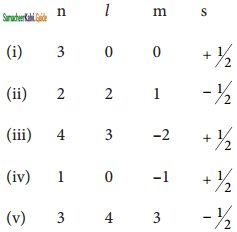Which of the following sets of quantum numbers is not possible?
(a) (i), (ii) and (iv)
(b) (ii), (iv) and (v)
(c) (i) and (iii)
(d) (ii), (iii) and (iv)
(b) (ii), (iv) and (v)

Question 18.
How many electrons in an atom with atomic number 105 can have (n + l) = 8?
(a) 30
(b) 17
(c) 15
(d) unpredictable
(b) 17

Question 19.
Electron density in the yz plane of 3dx2 – y2 orbital is
(a) zero
(b) 0.50
(c) 0.75
(d) 0.90
(a) zero

Question 20.
If uncertainty in position and momentum are equal, then minimum uncertainty in velocity is
(a) $$\frac{1}{m} \sqrt{\frac{h}{\pi}}$$

(b) $$\sqrt{\frac{\mathrm{h}}{\pi}}$$

(c) $$\frac{1}{2 m} \sqrt{\frac{h}{\pi}}$$

(d) $$\frac{\mathrm{h}}{4 \pi}$$
(c) $$\frac{1}{2 m} \sqrt{\frac{h}{\pi}}$$Question 21.
A macroscopic particle of mass 100 g and moving at a velocity of 100 cm s-1 will have a de Broglie wavelength of
(a) 6.6 × 10-29 cm
(b) 6.6 × 10-30 cm
(c) 6.6 × 10-31 cm
(d) 6.6 × 10-32 cm
(c) 6.6 × 10-31 cm

Question 22.
The ratio of de Brogue wavelengths of a deuterium atom to that of an α – particle, when the velocity of the former is five times greater than that of later, is
(a) 4
(b) 0.2
(c) 2.5
(d) 0.4
(d) 0.4

Question 23.
The energy of an electron in the 3rd orbit of a hydrogen atom is -E. The energy of an electron in the first orbit will be
(a)-3E
(b) -E/3
(c) -E/9
(d) -9E
(d) -9E

Question 24.
Time independent Schnodinger wave equation is
(a) Hψ = Eψ
(b) ∆2ψ + 8π2m(E + V)ψ
(c) $$\frac{\partial^{2} \psi}{\partial x^{2}}+\frac{\partial^{2} \psi}{\partial y^{2}}+\frac{\partial^{2} \psi}{\partial z^{2}}+\frac{2 m}{h^{2}}(\mathrm{E}-\mathrm{V}) \psi=0$$
(d) all of these
(a) Hψ = Eψ

Question 25.
Which of the following does not represent the mathematical expression for the Heisenberg uncertainty principle?
(a) ∆x . ∆p ≥ $$\frac{h}{4}$$
(b) ∆x . ∆v ≥ $$\frac{h}{4 \pi m}$$
(c) ∆E . ∆t ≥ $$\frac{h}{4 \pi}$$
(d) ∆E . ∆x ≥ $$\frac{h}{4 \pi}$$
(d) ∆E . ∆x ≥ $$\frac{h}{4 \pi}$$II. Write brief answers to the following questions:

Question 26.
Which quantum number reveals information about the shape, energy, orientation, and size of orbitals?
Magnetic quantum numbers reveal information about the shape, energy, orientation, and size of orbitals.

Question 27.
How many orbitals are possible for n = 4?
When n = 0, l = 0, 1,2 and 3. Hence, there are four subshells namely, s, p, d and f
l = 0, m1 = 0; one 4s orbital, l = 1, m = -1, 0, +1; three 4p orbitals,
l = 2, m1 = – 2, -1, 0, +1, +2; five 4d orbitals and
l = 3, m1 = – 3, -2, -1, 0, 1, 2, 3; seven 4f orbitals. Hence, the number of possible orbitals when n = 4 are sixteen.

Question 28.
How many radial nodes for 25, 4p, 5d and 4f orbitals exhibit? How many angular nodes?
The number of radial nodes is equal to (n – l – 1) and angular nodes is l.

 Orbital N l Radial node (n – l – 1) Angular node, l 2s 2 0 1 0 4p 4 1 2 1 5d 5 2 2 2 4f 4 3 0 3

The number of radial nodes for 2s, 4p, 5d, and 4f orbitals are respectively 1,2,2 and 0 and the number of angular nodes for 2s, 4p, 5d, and 4f orbitals respectively are 0, 1, 2, and 3.

Question 29.
The stabilization of a half-filled d – orbital is more pronounced than that of the p-orbital. Why?
The exactly half-filled orbitals have greater stability. The reason for their stability are –

1. symmetry
2. exchange energy.

(1) Symmetry: The half-filled orbitals are more symmetrical than partially filled orbitals and this symmetry leads to greater stability.

(2) Exchange energy: The electrons with the same spin in the different orbitals of the same sub-shell can exchange their position. Each such exchange releases energy and this is known as exchange energy. Greater the number of exchanges, the greater the exchange energy, and hence greater the stability. In d-orbital, 10 exchanges are possible but in p-orbital 6 exchanges are possible. So, d – orbital with 5 unpaired electrons (10 exchanges)n i.e. half-filled is more stable than p – orbital with 3 unpaired electrons (6 exchanges).

Question 30.
Consider the following electronic arrangements for the d5 configuration.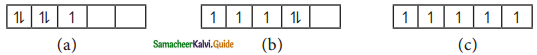(i) Which of these represents the ground state?
(ii) Which configuration has the maximum exchange energy?
(i) The ground state electronic configuration is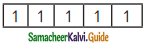(ii) The configuration has the maximum exchange energy isQuestion 31.
State and explain Paull’s exclusion principle.
Pauli’s exclusion principle states that “No two electrons in an atom can have the same set of values of all four quantum numbers”.
Illustration: H(Z = 1) 1s1.
One electron is present in hydrogen atom, the four quantum numbers are n = 1, l = 0, m = 0 and s = + $$\frac {1}{2}$$. For helium Z = 2. He: 1s2. In this one electron has the quantum number same as that of hydrogen, n = 1,l = 0, m = 0 and s = +½ For other electron, fourth quantum number is different, i.e. n = 1, l = 0, m = 0 and s = – ½.

Question 32.
Define orbital. What are ‘n’ and ‘l’ values for 3px and 4d x2 – y2 electron?
Orbital is a three-dimensional space in which the probability of finding the electron is maximum. The values of ‘n’ and ‘l’ for 3px orbital are n = 3 and l = 1, 4d x2 – y2 orbital are n = 4 and l = 2.

Question 33.
Explain briefly the time-independent Schrodinger wave equation.
Erwin Schrodinger expressed the wave nature of electrons in terms of a differential equation. This equation determines the change of wave function in space depending on the field of force in which the electron moves. The time-independent Schrodinger equation can be expressed as
Hψ = Eψ, where H is called Hamiltonian operator, ψ is the wave function and is a function of position coordinates of the particle and is denoted as ψ(x, y, z), E is the energy of the system.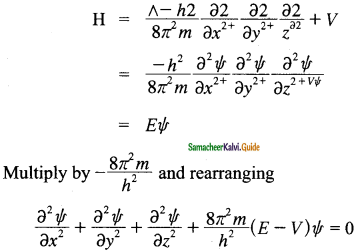The above Schrodinger wave equation does not contain time as a variable and is referred to as time-independent Schrodinger wave equation. This equation can be solved only for certain values of E, the total energy, i.e., the energy of the system is quantized. The permitted total energy values are called eigenvalues and corresponding wave functions represent the atomic orbitals.

Question 34.
Calculate the uncertainty in position of an electron, if ∆v = 0.1 % and υ = 2.2 × 106 ms-1.
Heisenberg’s Uncertainty Principle is ∆x. ∆v > h/4πm.
Given:
∆v = 0.1%,
υ = 22 × 106 ms-1.
h = 6.626 × 10-34 kgm2s-1 .
m = 9.1 X 10 21 kg.
∆v = $$\frac{0.1 \times 2.2 \times 10^{6} \mathrm{~ms}^{-1}}{100}$$

= 2.2 × 103 ms-1
Uncertainty in position,
∆x ≥ $$\frac{h}{4 \pi m}$$
∆x ≥ $$\frac{6.626 \times 10^{-34} \mathrm{kgm}^{2} \mathrm{~s}^{-1}}{9.1 \times 10^{-31} \mathrm{~kg} . \times 2.2 \times 10^{3} \mathrm{~ms}^{-1}}$$

∆x ≥ 2.64 × 10-8 m.

Question 35.
Determine the values of all the four quantum numbers of the 8th electron in the O – atom and 15th electron in the Cl atom and the last electron in Chromium.
(1) O (Z = 8) 1s2 2s2 2px2 2py1 2pz1
Four quantum numbers for 2px1 electron in oxygen atom:
n = principal quantum number = 2
l = azimuthal quantum number =1
m = magnetic quantum number =+1
s = spin quantum number = +$$\frac {1}{2}$$

(2) Cl (Z = 17) 1s2 2s2 2p6 3s2 3px2 3py2 3pz1
Four quantum numbers for 15th electron in chlorine atom:
n = 3, l = 1, m = 0, s = + ½

(3) Cr (Z = 24) 1s2 2s2 2p2 3s2 3p2 3d2 4s1
n = 3, l = 2, m = +2, s = + ½Question 36.
The quantum mechanical treatment of the hydrogen atom gives the energy value:
En = –$$\frac{-13.6}{n^{2}}$$ eV/atom
(i) Use this expression to find ∆E between n = 3 and n = 4.
(ii) Calculate the wavelength corresponding to the above transition.
Energy of the electron in the nth orbit is
En = –$$\frac{-13.6}{n^{2}}$$eV/atom.

When n = 3,
E3 = $$\frac{-13.6 \mathrm{eV} / \text { atom }=-1.51 \mathrm{eV} / \text { atom }}{9}$$

When n = 4,
E4 = $$\frac{-13.6 \mathrm{eV} / \text { atom }=-0.85 \mathrm{eV} / \text { atom }}{16}$$

∆E = (E4 – E3) = (-0.85) – (-1.51) = 0.66 eV/atom
Wavelength corresponding to this transition,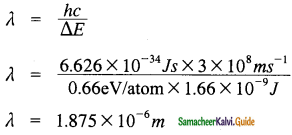Question 37.
How fast must a 54g tennis ball travel in order to have a de Broglie wavelength that is equal to that of a photon of green light 5400 Å?
de Broglie wavelength,
λ = $$\frac{h}{m v}$$
Given:
de Broglie wavelength, λ = 5400 Å and mass, m = 54 g.
Velocity of the tennis ball
v = $$\frac{h}{m \lambda}$$

v = $$\frac{6.626 \times 10^{-34} J s}{54 \times 10^{-3} k g \times 5400 \times 10^{-10} m}$$

v = 2.27 × 10-26 ms-1

Question 38.
For each of the following, give the sub level designation, the allowable m values and the number of orbitals,
(i) n = 1, l = 2
(ii) n = 5, l = 3
(iii) n = 7, l = 0Question 39.
Give the electronic configuration of Mn2+ and Cr3+.
1. Mn (Z = 25)
Mn → Mn2+ + 2e
Mn2+ electronic configuration is 1s 1s2 2s2 2p6 3s2 3p6 3d5

2. Cr (Z = 24)
Cr → Cr3+ + 3e
Cr3+ electronic configuration is Is2 2s2 2p6 3s23p6 3d3

Question 40.
Describe the Aufbau principle.
Aufbau Principle states that “In the ground state of the atoms, the orbitals are filled in the order of their increasing energies”. That is the electrons first occupy the lowest energy orbital available to them. Once the lower energy orbitals are completely filled, then the electrons enter the next higher energy orbitals. The order of filling of various orbitals as per the Aufbau principle is given in the figure. which is in accordance with the (n + l) rule.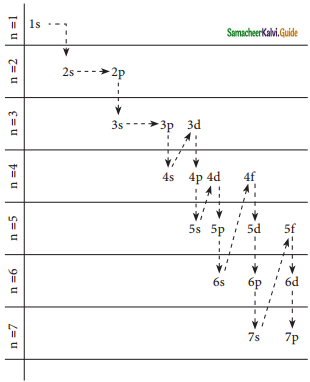Question 41.
An atom of an element contains 35 electrons and 45 neutrons. Deduce
(i) the number of protons
(ii) the electronic configuration for the element
(iii) All the four quantum numbers for the last electron.
(i) Atomic Number of the element, z = No. of protons or No. of electrons. = 35
Mass number of the element, A = No. of protons + No of neutrons = 35 + 45 = 80
Number of Protons = 80 – 45 = 35.

(ii) Electronic configuration of the element (z = 35)
1s2 2s2 2p6 3s2 3p6 4s2 3d10 4p5

(iii) Quantum number for the last electron (4pz),
n = 4, l = 1, m = +1, or -1 s = +1/2

Question 42.
Show that the circumference of the Bohr orbit for the hydrogen atom is an integral multiple of the de Broglie wavelength associated with the electron revolving around the nucleus.
According to the de Broglie concept, the electron that revolves around the nucleus exhibits both particle and wave character. In order for the electron wave to exist in phase, the circumference of the orbit should be an integral multiple of the wavelength of the electron wave, Otherwise, the electron wave is out of phase.
Circumference of the orbit = nλ
2πr = nλ
2πr = $$\frac{n h}{m v}$$
Rearranging,
mvr = $$\frac{n h}{m v}$$
Angular momentum = $$\frac{n h}{2 \pi}$$
The above equation was already predicted by Bohr.
Hence, de Broglie and Bohr’s concepts are in agreement with each other.

Question 43.
Calculate the energy required for the process.
He+(g) → He2+(g) + e
The ionization energy for the H atom in its ground state is — 13.6 eV/atom.
He+(g) → He2+(g) + e and
En = – 13.6z2/n2
El = – $$\frac{13.6(2)^{2}}{(1)^{2}}$$ = -56.4 eV

E = $$\frac{-13.6(2)^{2}}{(\infty)^{2}}$$ = 0

Required energy for the given process is,
E – El = 0 – (-56.4) = 56.4 eV.

Question 44.
An ion with mass number 37 possesses unit negative charge. It the ion contains 11.1% more neutrons than electrons. Find the symbol of the ion.
Let the number of electrons in an ion = x
number of neutrons = n = x + $$\frac{11.1}{100}$$ eV = 1.111 x
(As the number of neutrons is 11.1% more than the number of electrons)
In the neutral of the atom, a number of electrons.
e = x – 1 (as the ion carries -1 charge)
Similarly number of protons = P = x – 1
Number of protons + number of neutrons = mass number = 37
(x – 1) + 1.111 x = 37 .
2.111 x = 37 +1
2.111 x = 38
x = $$\frac{38}{2.111}$$ = 18.009 = 18
∴ Number of protons = atomic number – 1 = 18-1 = 17
∴ The symbol of the ion = $$_{17}^{37} \mathrm{Cl}$$.

Question 45.
The Li2+ ion is a hydrogen-like ¡on that can be described by the Bohr model. Calculate the Bohr radius of the third orbit and calculate the energy of an electron in 4th orbit.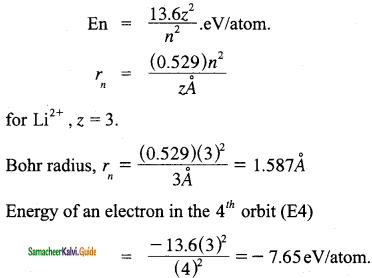Question 46.
Protons can be accelerated in particle accelerators. Calculate the wavelength (in Å) of such accelerated proton moving at 2.85 × 108ms-1 (mass of proton is 1.673 × 10-27 kg).
Given:
velocity, v = 2.85 × 108ms-1.
mass, m = 1.673 × 10-27 kg
λ = $$\frac{h}{m v}$$

λ = $$\frac{6.626 \times 10^{-34} \mathrm{kgms}^{-1}}{1.673 \times 10-27 \mathrm{~kg} \times 2-85 \times 10^{8} \mathrm{~ms}^{-1}}$$

λ = 1.389 × 10-8 Å

Question 47.
What is the de Broglie wavelength (in cm) of a 160g cricket ball travelling at 140 Km hr-1.
Given:
velocity, v = 140 km/hr. = $$\frac{140 \times 10^{3}}{60 \times 60 \mathrm{~ms}^{-1}}$$

mass, m = 160g = 160 × 10-3 kg
λ = $$\frac{h}{m v}$$
λ =$$\frac{6.626 \times 10^{-34} \mathrm{kgm}^{2} \mathrm{~s}^{-\mathrm{le}}}{160 \times 10^{-3} \mathrm{~kg} \times 3.88 \mathrm{~ms}^{-1}}$$

λ = 1.605 × 10-34 m.

Question 48.
Suppose that the uncertainty in determining the position of an electron in an orbit is 0.6 Å. What is the uncertainty in its momentum?
Heisenberg Uncertainity Principle is ∆x . ∆p ≥ $$\frac{h}{4 \pi}$$
Given:
∆x = 0.6 Å = 0.6 × 10-10 m
h = 6.626 × 10-34 kgm2s-1
Uncertainity in momentum,
∆p ≥ $$\frac{h}{4 \pi \Delta x}$$

∆p ≥ $$\frac{6.626 \times 10^{-34} \mathrm{kgm}^{2} \mathrm{~s}^{-1}}{4 \times 3.14 \times 0.6 \times 10^{-10} \mathrm{~m}}$$

∆p ≥ 8.8 × 10-25 kgms-1

Question 49.
Show that if the measurement of the uncertainty in the location of the particle is equal to its de Broglie wavelength, the minimum uncertainty in its velocity (∆V) is equal to 1/4π of its velocity(V)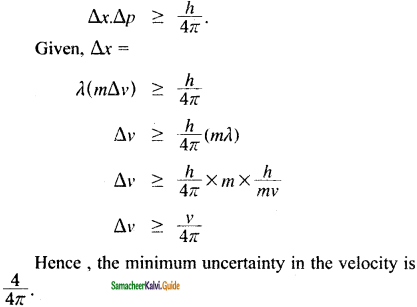Question 50.
What is the de Brogue wave length of an electron, which is accelerated from the rest, through a potential difference of 100V?
Potential difference = 100 V = 100 × 106 × 10-19 J
λ = $$\frac{h}{\sqrt{2} \text { mev }}$$

λ = $$\frac{6.626 \times 10^{-34} \mathrm{Kgm}^{2} \mathrm{~s}}{\sqrt{2} \times 9.1 \times 10^{-31} \mathrm{~kg} \times 100 \times 1.6 \times 10^{-19} \mathrm{~J}}$$

λ = 1.22 × 10-10Question 51.
Identify the missing quantum numbers and the sub energy level.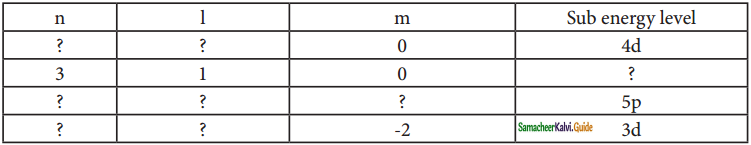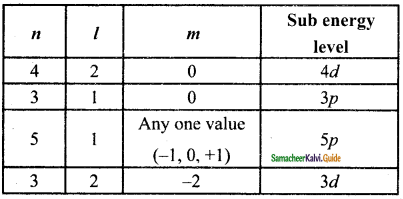### 11th Chemistry Guide Quantum Mechanical Model of Atom Additional Questions and Answers

I. Choose the best Answer:

Question 1.
The angular momentum of the electron in the nth orbit is
(a) $$\frac{\pi h}{2 n}$$

(b) $$\frac{2 n h}{\pi}$$

(c) $$\frac{n h}{2 \pi}$$

(d) $$\frac{2 \pi}{n h}$$
(c) $$\frac{n h}{2 \pi}$$

Question 2.
The frequency of radiation emitted when an electron jumps from higher energy state (E2) to a lower energy state (E1) is given by
(a) v = $$\frac{\left(E_{2}+E_{1}\right)}{h}$$

(b) v = $$\frac{\left(E_{1}+E_{2}\right)}{h}$$

(c) v = $$\frac{\left(E_{1}-E_{2}\right)}{h}$$

(d) v = $$\frac{\left(E_{2}-E_{1}\right)}{h}$$
(d) v = $$\frac{\left(E_{2}-E_{1}\right)}{h}$$

Question 3.
Splitting of spectral lines in the presence of a magnetic field is called
(a) Zeeman effect
(b) Stark effect
(e) shielding effect
(d) Compton effect
(a) Zeeman effect

Question 4.
Which one of the following has zero rest mass?
(a) electron
(b) proton
(c) neutron
(d) photon
(d) photon

Question 5.
For a microscopic particle such as an electron, the mass is of the order of
(a) 10-29 kg
(b) 10-31 kg
(c) 1031 kg
(d) 10-30 kg
(b) 10-31 kgQuestion 6.
Which one of the following has insignificant de Broglie wavelength?
(a) electron
(b) proton
(e) neutron
(d) iron ball
(d) iron ball

Question 7.
Which of the following statements are true about de Broglie wavelength?
(i) The particle travels at a speed much higher than the speed of light.
(ii) The particle can have high linear momentum.
(iii) The mass of the particle is of the order of 10-30 kg.
(iv) The particle travels at speed much less than the speed of light.
(a) (i) and (ii)
(b) (iii) and (iv)
(c) (i) and (iii)
(d) (ii) and (iv)
(b) (iii) and (iv)

Question 8.
The correct mathematical expression/s for Heisenberg Uncertainty principle is
(i) ∆x . ∆p ≥ $$\frac{h}{4 \pi}$$

(ii) ∆x . ∆p ≥ $$\frac{h}{4 \pi m}$$

(iii) ∆x . ∆v ≥ $$\frac{h}{4 \pi m}$$

(iv) ∆x . ∆v ≥ $$\frac{h}{4 \pi}$$
(a) (i) and (ii)
(b) (ii) and(iv)
(c) (i) and (iii)
(d) (ii) and (iii)
(c) (i) and (iii)

Question 9.
The wave nature of electron was experimentally confirmed by
(a) Louis de Broglie
(b) Davisson and German
(c) Schrodinger
(d) Niels Bohr
(b) Davisson and German

Question 10.
The term ‘ ψ ’ in the Schrodinger equation is
(a) Eigenvalue
(b) Hamiltonian operator
(c) Wave function
(d) all of these
(c) Wave functionQuestion 11.
The permitted total energy values in the Schrodinger equation are called
(a) eigenvalues
(b) eigen functions
(c) wave functions
(d) Hamiltonian operator
(a) eigenvalues

Question 12.
_______ is a three-dimensional space in which the probability of finding the electron is maximum.
(a) orbit
(b) Orbital
(c) wave function
(d) eigenvalue
(b) Orbital

Question 13.
Which of the following has always positive value?
(a) ψ
(b) ψ2
(c) both ψ and ψ2
(d) ψ3
(b) ψ2

Question 14.
The maximum number of electrons that can be accommodated in M shell is
(a) 8
(b) 32
(c) 16
(d) 18
(d) 18

Question 15.
The maximum number of electrons that can be accommodated in a given subshell is
(a) (2l + 1)
(b) 4l + 2
(c) l + 2
(d) 2(l + 1)
(b) 4l + 2Question 16.
The region where the probability density function reduces to zero is called
(a) wave function
(b) orbital
(c) nodal surface
(d) probability density region
(c) nodal surface

Question 17.
Number of subshells and electrons associated with n = 4 respectively are
(a) 4, 16
(b) 32, 64
(c) 16, 32
(d) 8, 16
(c) 16, 32

Question 18.
The radial wave function in the ψ(r, θ, φ) = R(r), f(θ), g(φ) is
(a) f(θ)
(b) g(φ)
(c) R(r)
(d) f(θ) and g(φ)
(c) R(r)

Question 19.
The plot of _______ shows the maximum probability that occurs at a distance of 0.52 Å from the nucleus.
(a) 4π2r2 vs ψ2
(b) 4π2 r2 ψ2 vs r2
(c) 4π2 ψ2 vs r
(d) 4π2 ψ2 vs r2
(d) 4π2 ψ2 vs r2

Question 20.
The number of radial nodes for a 3s orbital is
(a) 3
(b) 2
(c) 1
(d) 0
(b) 2Question 21.
The number radial nodes for a ‘nd’ orbital is
(a) (n – 1)
(b) (n – l)
(c) (n – l + 1)
(d) (n – l – 1)
(d) (n – l – 1)

Question 22.
The order of the effective nuclear charge felt by an electron in an orbital within a shell is
(a) s < p < d < f
(b) s > p > d > f
(c) s < p ≈ d < f
(d) s ≈ p > d ≈ f
(b) s > p > d > f

Question 23.
Which of the following sequences shows the correct increasing order of energy’?
(a) 3s, 3p, 4s, 3d, 4p, 5s, 4d, 5p
(b) 3s, 3p, 4s, 4p, 3d, 5s, 4d, 5p
(c) 3s, 3p, 4s, 4p, 3d, 5s, 5p, 4d
(d) 3s, 3p, 4s, 4p, 3d, 4d, 5s, 5p
(a) 3s, 3p, 4s, 3d, 4p, 5s, 4d, 5p

Question 24.
The orbital with following quantum numbers (i) n = 4,1 = 3 and (ii) n = 3 and 1 = 2 are
(a) 3d, 4f
(b) 3d, 4d
(c) 3f, 4f
(d) 3p, 4f
(c) 3f, 4f

Question 25.
The energy of one photon of a beam of light with wavelength 3.31 × 10-6 m is
(a) 6 × 10-20 J
(b) 2 × 10-20 J
(c) 6 × 10-21 J
(d) 2 × 10-21 J
(a) 6 × 10-20 JQuestion 26.
The ratio of energies of two radiations with wavelengths of 300 nm and 900 nm is
(a) 1 : 3
(b) 2 : 1
(c) 3 : 1
(d) 1 : 2
(c) 3 : 1

Question 27.
The momentum of a particle which has a wavelength of 6.62 Å is (in kg ms-1)
(a) 10-20
(b) 10-22
(c) 10-34
(d) 10-24
(d) 10-24

Question 28.
If the energy difference between the ground state of an atom and its excited state is 3.31 × 10-19 J, the wavelength of the photon required to produce this transition is
(a) 3 × 10-9 m
(b) 6 × 10-7 m
(c) 3 × 10-8 m
(d) 6 × 10-6 m
(b) 6 × 10-7 m

Question 29.
The spectrum of He+ is similar to
(a) Li+
(b) H+
(c) He
(d) Li2+
(c) He

Question 30.
An atom has two electrons in the K shell, eight electrons in the L shell, and eight electrons in the M shell. The number of ‘s’ electrons present in that element is
(a) 18
(b) 10
(c) 6
(d) 4
(c) 6Question 31.
The atomic number of an element ‘A’ is 25. How many electrons are present in the third shell of the element in its A3+ state?
(a) 10
(b) 12
(c) 15
(d) 4
(b) 12

Question 32.
Which of the following sets of quantum numbers are not possible?(a) (i), (iii) and (iv)
(b) (i), (iv) and (v)
(c) (iii), (iv) and (v)
(d) (i), (ii) and (iii)
(c) (iii), (iv) and (v)

Question 33.
ψ2 is always
(a) negative
(b) positive
(c) either positive or negative
(d) none of these
(b) positive

Question 34.
Which of the following sets of quantum numbers represents the highest energy of an atom?
(a) n = 4, l = 1, m = 0, s = +1/2
(b) n = 3, l = 0, m = 0, s = +1/2
(c) n = 3, l = 0, m = 1, s = +1/2
(d) n = 4, l = 0, m = 0, s = +1/2
(a) n = 4, l = 1, m = 0, s = +1/2

Question 35.
The number of possible exchanges for [Ar] 3d4 4s2 configuration is
(a) 10
(b) 6
(c) 4
(d) 1
(b) 6II. Very Short Question and Answers (2 Marks):

Question 1.
Write a note about J.J. Thomson’s atomic model.

• J.J. Thomson’s cathode ray experiment revealed that atoms consist of negatively charged particles called electrons.
• He proposed that an atom is a positively charged sphere in which the electrons are embedded like the seeds in the watermelon.

Question 2.
What is the Zeeman effect?
The splitting of spectral lines in the presence of a magnetic field is called the Zeeman effect.

Question 3.
What is Stark effect?
The splitting of spectral lines in the presence of electric field is called Stark effect.

Question 4.
Write the limitation of Bohr’s postulates?
Bohr’s postulates are applicable to one electron speciesvsuch as H, He+ and Li2+ etc.,

Question 5.
Write the formulae for the radius and energy of electron in the nth orbit of electron.
Radius of electron in the nth orbit,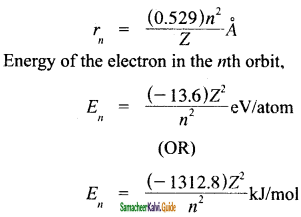Question 6.
Write de Broglie equation and explain the terms in it.
de Beoglie equation is λ = $$\frac{h}{m v}$$
where λ = de Broglie wavelength of matter waves, m = mass of the particle and v = velocity.

Question 7.
What are quantum numbers?

• The electron in an atom can be characterized by a set of four quantum numbers, namely principal quantum number (n), azimuthal quantum number (l), magnetic quantum number (m), and spin quantum number (s).
• When the Schrodinger equation is solved for a wave function T, the solution contains the first three quantum numbers n, l, and m.
• The fourth quantum number arises due to the spinning of the electron about its own axis.

Question 8.
State Heisenberg Uncertainty principle.
It is impossible to accurately determine both the position as well as the momentum of a microscopic particle simultaneously.

Question 9.
What is classical mechanics?
The motion of objects that we come across in our daily life can be well described based on Newton’s law of motion is called classical mechanics.

Question 10.
What is the limitation of classical mechanics?
Classical mechanics does not consider the dual nature of the matter which is significant for microscopic particles. As a consequence, it fails to explain the motion of microscopic particles.Question 11.
What is quantum mechanics?
Based on Heisenberg’s principle and the dual I nature of the microscopic particles, a new mechanics I developed to study the motion of microscopic particles is called quantum mechanics.

Question 12.
What is Schrodinger’s equation?
Erwin Schrodinger expressed the wave nature of electron in terms of the differential equation is called Schrodinger equation. This equation determines the change of wave equation in space depending on the field of force in which the electron moves.

Question 13.
Write the Schrodinger equation in terms of the operator?
The time-independent Schrodinger equation can be expressed as Hψ = Eψ
where H is called Hamiltonian operator, ψ is the wave function and E is the energy of the system.

Question 14.
Write the Schrodinger equation in terms of a differential equation?
The time independent Schrodinger equation in terms of a differential equation is
$$\frac{\partial^{2} \Psi}{\partial x^{2}}+\frac{\partial^{2} \Psi}{\partial y^{2}}+\frac{\partial^{2} \Psi}{\partial z^{2}}+\frac{8 \pi^{2} m}{h^{2}}(\mathrm{E}-\mathrm{V}) \Psi=0$$

Question 15.
What is a Hamiltonian operator?
The total energy operator is called Hamiltonian operator and it can be expressed as
Ĥ = $\frac{-h^{2}}{8 \pi^{2} m}\left(\frac{\partial^{2}}{\partial x^{2}}+\frac{\partial^{2}}{\partial y^{2}}+\frac{\partial^{2}}{\partial z^{2}}\right)+V$Question 16.
Define an orbital.
Orbital is a three-dimensional space in which the probability of finding the electron is maximum.

Question 17.
Calculate the total number of angular nodes and radial nodes present in 4p and 4d orbitals.
1. For 4p orbital:
Number of angular nodes = l
For 4p orbital 7 = l
Number of angular nodes = l
Number of radial nodes = n – l – 1 = 4 -1 -1 = 2
Total number of nodes = n -1 = 4 – 1 = 3
1 angular node and 2 radial nodes.

2. For 4d orbital:
Number of angular nodes = l
For 4d orbital l = 2
Number of angular nodes = 2
Number of radial nodes = n – l – 1 = 4 – 2 – 1 = 1
Total number of nodes = n – l = 4 – l = 3
1 radial nodes and 2 angular node.

Question 18.
The energies of the same orbital decrease with an increase in the atomic number. Justify this statement.
The energy of the 2s orbital of a hydrogen atom is greater than that of 2s orbital of lithium and that of lithium is greater than that of sodium and so on because of H (Z =1), Li (Z = 3), and Na (Z = 11). When the atomic number increases, the energies of the same orbital decrease. E2s(H) > E2s(Li) > E2s(Na) > E2s(K) ………….

Question 19.
What is the principal quantum number?
The principal quantum number represents the energy level in which an electron revolving around the nucleus and is denoted by the symbol ‘n’.

Question 20.
What is a nodal surface or a radial node?
The region where this probability density function reduces to zero is called nodal surface or a radial node.Question 21.
What is the significance of the solution to the Schrodinger equation?
The solution to the Schrodinger equation gives the permitted energy values called eigenvalues and the wave functions corresponding to the eigenvalues are called atomic orbitals.

Question 22.
What are radial and angular wave functions?
The solution of the Schrodinger wave equation for one electron system can be expressed in spherical polar coordinates as ψ(r, θ, φ) = R(r). f(θ). g(φ) Where R(r) is called a radial wave function, f(θ) and g(φ) are called angular wave functions.

Question 23.
What is the ground state?
The electron present in the lowest energy state is called the ground state.

Question 24.
State (n + l) rule.
The lower the value of (n + l) for an orbital, the lower is its energy. If two orbitals have the same value of (n + l), the orbital with lower value of ‘n’ will have the lower energy.III. Short Question and Answers (3 Marks):

Question 1.
What are the conclusions of Rutherford’s α – rays scattering experiment?

• Rutherford bombarded a thin gold foil with a stream of fast-moving α – particles.
• It was observed that most of the a-particles passed through the foil.
• Some of them were deflected through a small angle.
• Very few α- particles were reflected back by 180°.
• Based on these observations, he proposed that in an atom, there is a tiny positively charged nucleus and the electrons are moving around the nucleus with high speed.

Question 2.
Write the assumptions of Bohr’s atom model.
Bohr’s atom model is based on the following assumptions:
(i) The energies of electrons are quantized.
(ii) The electron is revolving around the nucleus in a certain fixed circular path called stationary orbit.
(iii) Electron can revolve only in those orbitsJn which the angular momentum {mvr) of the electron must be equal to an integral multiple of $$\frac{h}{2 \pi}$$
i,e., mvr = $$\frac{n h}{2 \pi}$$, where n = 1, 2, 3, … etc.,
(iv) As long as an electron revolves in the fixed orbit, it does lose its energy. However, when an electron jumps from higher energy state (E2) to a lower energy state (E1), the excess energy is emitted as radiation.
The frequency of the emitted radiation is (E2 – E1) = hυ.

Frequency, υ = $$\frac{\left(E_{2}-E_{1}\right)}{h}$$
Conversely, when suitable energy is supplied to an electron, it will jump from lower energy orbit to a higher energy orbit.

Question 3.
Explain Davisson and Germer’s experiment.

• The wave nature of electrons was experimentally confirmed by Davisson and Germer.
• They allowed the accelerated beam of electrons to fall on a nickel crystal and recorded the diffraction pattern.
• The resultant diffraction pattern is similar to the X-ray diffraction pattern.
• The finding of wave nature of electron leads to the development of various experimental techniques such as electron microscope, low energy electron diffraction, etc.

Question 4.
Show that de Broglie and Bohr’s concepts are in agreement with each other.
According to the de Broglie concept, the electron that revolves around the nucleus exhibits both particle and wave character. In order for the electron wave to exist in phase, the circumference of the orbit should be an integral multiple of the wavelength of the electron wave, Otherwise, the electron wave is out of phase.
Circumference of the orbit = nλ
2πr = nλ
2πr = $$\frac{n h}{m v}$$
Rearranging,
mvr = $$\frac{n h}{2 \pi}$$
Angular momentum = $$\frac{n h}{2 \pi}$$
The above equation was already predicted by Bohr. Hence, de Broglie and Bohr’s concepts are in agreement with each other.

Question 5.
Write a note about the principal quantum number.

• The principal quantum number represents the energy level in which an electron revolves around the nucleus and is denoted by the symbol ‘n’.
• The ‘n’ can have the values 1, 2, 3,… n = 1 represents K shell; n=2 represents L shell and n = 3, 4, 5 represent the M, N, O shells, respectively.
• The maximum number of electrons that can be accommodated in a given shell is 2n2.
• ‘n’ gives the energy of the electron,

En = $$\frac{(-1312.8) Z^{2}}{n^{2}}$$ KJ mol-1 and the distance of the electron from the nucleus is given by rn = $$\frac{(-0.529) n^{2}}{Z}$$ A.Question 6.
Write the significance of principle quantum number.
Principle quantum number represents the energy level in which electron revolves around the nucleus and is denoted by the symbol ‘n’.
(i) The ‘n’ can have the values 1, 2, 3, … n = 1 represent K shell: n = 2 represents L shell and n = 3, 4, 5 represent the M, N, O shells respectively.
(ii) The maximum number of electrons that can be accommodated in a given shell, is 2n2.
(iii) ‘n’ gives the energy of the electron,
En = $$\frac{(-1312.8) Z^{2}}{n^{2} k J m o l^{-1}}$$

and the distance of the electron from the nucleus is given by
rn = $$\frac{(0.529) n^{2}}{Z}$$ Å.

Question 7.
Write notes on Azimuthal Quantum number.
(i) Azimuthal quantum number is represented by the letter ‘l’ and can take integral values from zero to (n – l), where n is the principle quantum number.
(ii) Each l value represents a subshell. l = 0, 1,2, 3 and 4 represents the s, p, d, f and g orbitals respectively.
(iii) The maximum number of electrons that can be accommodated in a given subshell is 2 (2l + 1).
(iv) It is used to calculate the orbital angular momentum using the expression
Angular momentum = $$\frac{\sqrt{l(l+1) h}}{2 \pi}$$

Question 8.
Write the significance of magnetic quantum numbers.
(i) Spin quantum number is denoted by the letter ‘ml’. It takes integral values ranging from -l to +l through 0. i.e., if l = 1: m = -1, 0 and +1.
(ii) Different values of m for a given l value, represent different orientations of orbitals in space.
(iii) The Zeeman effect provides the experimental justification for this quantum number.
(iv) The magnitude of the angular momentum is determined by the quantum number ‘l’ while its direction is given by magnetic quantum number.

Question 9.
Write notes on spin quantum number.
(i) The spin quantum number represents the spin of the electron and is denoted by the letter ‘ms‘.
(ii) The electron in an atom revolves not only around the nucleus but also spins. It is usual to write this as an electron spins about its own axis either in a clockwise direction or in an anti-clockwise direction. The visualization is not true. However, spin is to be understood as representing a property that revealed itself in magnetic fields.
(iii) Corresponding to the clockwise and anti¬clockwise spinning of the electron, a maximum of two values are possible for this quantum number.
(iv) The values of ‘ms‘ is equal to -1/2 and +1/2.

Question 10.
Write the significance of ψ and ψ2.
The wave function ψ has no physical meaning and the square of the wave function |ψ|2 is related to the probability of finding the electrons within a given volume of space. |ψ|2 varies with the distance from nucleus (radial distribution of the probability) and the direction from the nucleus (angular distribution of the probability).Question 11.
Show that the probability of finding the electron is independent of the direction from the nucleus.
The variation of the probability of locating the electron on a sphere with nucleus at its centre depends on the azimuthal quamtum number of the orbital in which the electron is present. For 1s orbital, l = 0, and m = 0.
f(θ) = $$\frac{1}{\sqrt{2}}$$ and g(φ) = $$\frac{1}{\sqrt{2 \pi}}$$

Therefore, the angular distribution function is equal to $$\frac{1}{2 \sqrt{\pi}}$$ i.e., it is independeent of the angle θ and φ. Hence, the probability of finding the electron is independent of the direction from the nucleus.

Question 12.
Sketch the shapes of 1s, 2s and 3s orbitals.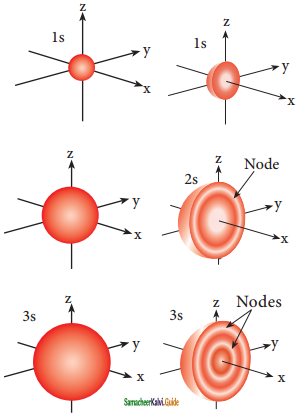Question 13.
Sketch and explain the shapes of p-orbitals.
The shape of the p-orbitais are shown in figure, For p orbitaIs, l = 1 and the corresponding m values are -1, 0 and +1. The three different ‘m’ values indicates that there are three different orientations possible for p orbitals. These orbitals are designated as px, py and pz and the angular distribution for these orbitals shows that the lobes are along the x, y and z axis respectively.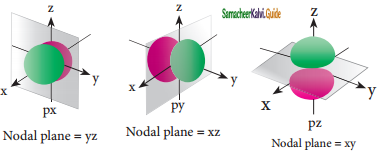Question 14.
What are ground and excited states?
The electron in the hydrogen atom occupies the ly orbital that has the lowest energy. This state is called ground state. When this electron gains some energy, it moves to the higher energy orbitals such as 2s, 2p etc., These states are called excited states.

Question 15.
Explain the significance of effective nuclear charge.
In a multi-electron atom, in addition to the electrostatic attractive force between the electron and nucleus, there exists a repulsive force among the electrons. These two forces are operating in the opposite direction. This results in the decrease in the nuclear force of attraction on electron.
The net charge experienced by the electron is called effective nuclear charge. The effective nuclear charge depends on the shape of the orbitals and it decreases with increase in azimuthal quantum number l. The order of the effective nuclear charge felt by a electron in an orbital within the given shell is s > p > d > l.

Greater the effective nuclear charge, greater is the stability of the orbital. Hence, within a given energy level, the energy of the orbitals are in the following order s < p < d <f.Question 16.
State and explain Hund’s rule.
Hund’s rule of maximum multiplicity states that- electron pairing in the degenerate orbitals does not take place until all the available orbitals contains one electron each. Consider the carbon atom which has six electrons. According to Aufbau principle, the electronic configuration is 1s2, 2s2, 2p2.
It can be represented as below,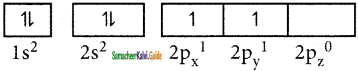In this case, in order to minimize the electron- electron repulsion, the sixth electron enters the unoccupied 2py orbital as per Hund’s rule, i.e., it does not get paired with the fifth electron already present in the 2px orbital.

IV. Long Question and Answers:

Question 1.
Derive de Broglie equation.
Albert Einstein proposed that light has dual nature. i.e., light photons behave both like a particle and as a wave. Louis de Broglie extended this concept and proposed that all forms of matter showed dual character. To quantify this relation, he derived an equation for the wave length of a matter wave. He combined the following two equations of energy of which one represents wave character (hυ) and the other represents the particle nature (mc2).
Planck’s quantum hypothesis is
E = hυ ………..(1)
Einstein’s mass-energy relationship,
E = mc2
From (1) and (2), hυ = mc2
$$\frac{h c}{\lambda}$$ = mc2
λ = $$\frac{h}{m c}$$
Equation (3) represents the wavelength of photons whose momentum is given by mc. For a particle of matter with mass ‘m’ and moving with a velocity ‘v’, the equation (3) can be written as
λ = $$\frac{h}{m v}$$ …………..(4)

The equation (4) is called de Broglie equation for matter waves and this is valid only when the particle travels at speeds much less than the speed of light. This equation implies that a moving particle can be considered as a wave and a wave can exhibit the properties of a particle.

For a particle with high linear momentum the wavelength will be so small and cannot be observed. For a microscopic particle such as an electron, the mass is of the order of 10-31 kg, hence the wavelength is much larger than the size of atom and it becomes significant.

Question 2.
Write the main features of the quantum mechanical model of atom.
(i) The energy of electrons in atoms is quantized.
(ii) The existence of quantized electronic energy levels is a direct result of the wave-like properties of electrons.
(iii) According to Heisenberg uncertainty principle, the exact position and momentum of an electron cannot be determined with absolute accuracy. As a consequence, quantum mechanics introduced the concept of orbital. Orbital is a three dimensional space in which the probability of finding the electron is maximum.
(iv) The solution of Schrodinger wave equation for the allowed energies of an atom gives the wave function, ψ, which represents an atomic orbital. The wave nature of electron present in an orbital can be well defined by the wave function ψ.
(v) The wave function, ψ, itself has no physical meaning. However, the probability of finding the electron in a small volume dxdydz around a point (x, y, z) is proportional to |ψ(x, y, z)|2 dxdydz. |ψ(x, y, z)|2 is known as probability density and is always positive.Question 3.
Describe the radial distribution function of 1s and 2s orbitals of hydrogen atom.
Consider a single electron of hydrogen atom in the ground state for which the quantum numbers are n = 1 and l = 0. i.e., it occupies l.y orbital. The plot R(r)2 versus r for is orbital given in figure.

The graph shows that as the distance between the electron and the nucleus decreases, the probability of finding the electron increases. At r = 0, the quantity R(r)2 is maximum. The maximum value for |ψ|2 is at the nucleus. However, probability of finding the electron in a given spherical shell around the nucleus is important. Let us consider the volume (dV) bounded by two spheres of radii r and r + dr.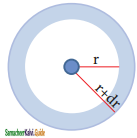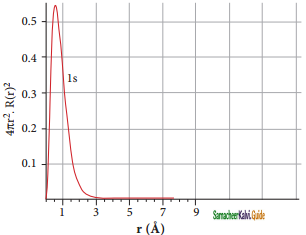The above plot shows that the maximum probability occurs at distance of 0.52 Å. from the nucleus. This is equal to the Bohr radius. It indicates that the maximum probability of finding the electron around the nucleus is at this distance. However, there is a probability to find the electron at other distances also. The radial distribution function of 2s, orbital of the hydrogen atom represented as follows.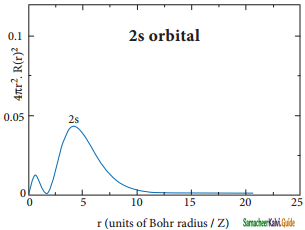Question 4.
Sketch and explain the shapes of d-orbitals.